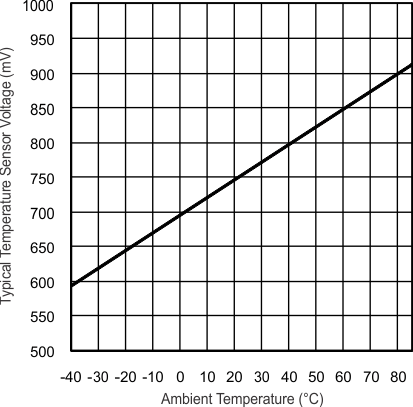JAJSG43B June   2012  – September 2018

PRODUCTION DATA.

1. 1デバイスの概要
2. 2改訂履歴
3. 3Device Comparison
4. 4Terminal Configuration and Functions
1. 4.1 Pin Diagrams
2. 4.2 Signal Descriptions
5. 5Specifications
6. 6Detailed Description
7. 7Applications, Implementation, and Layout
8. 8デバイスおよびドキュメントのサポート
9. 9メカニカル、パッケージ、および注文情報

• RGZ|48
• RGZ|48

5.39 REF, Built-In Reference

over recommended ranges of supply voltage and operating free-air temperature (unless otherwise noted)(1)
PARAMETER TEST CONDITIONS VCC MIN TYP MAX UNIT
VEREF+ Positive built-in reference voltage REFVSEL = \{2\} for 2.5 V,
REFON = REFOUT = 1
3 V 2.5 ±1.5% V
REFVSEL = \{1\} for 2 V,
REFON = REFOUT = 1
3 V 2.01 ±1.5%
REFVSEL = \{0\} for 1.5 V,
REFON = REFOUT = 1
2.2 V, 3 V 1.505 ±1.5%
AVCC(min) AVCC minimum voltage, Positive built-in reference active REFVSEL = \{0\} for 1.5 V 1.8 V
REFVSEL = \{1\} for 2 V 2.3
REFVSEL = \{2\} for 2.5 V 2.8
IREF+ Operating supply current into AVCC terminal(1) fADC10CLK = 5 MHz,
REFON = 1, REFBURST = 0,
REFVSEL = \{0\} for 1.5 V
3 V 15.5 19 µA
REFON = 1, REFBURST = 0,
REFVSEL = \{1\} for 2 V
3 V 18 24
REFON = 1, REFBURST = 0,
REFVSEL = \{2\} for 2.5 V
3 V 21 30
IREF+,REFOUT Operating supply current into AVCC terminal with REF output buffer enabled REFON = 1, REFOUT = 1,
REFBURST = 0
3 V 0.9 1.7 mA
IL(VREF+) Load-current regulation, VREF+ terminal(2) REFVSEL = \{0, 1, 2\},
ILoad,VREF+ = +10 µA or –1000 µA,
AVCC = AVCC(min) for each reference level, REFON = REFOUT = 1
2500 µV/mA
CVREF+ Capacitance at VREF+ terminals REFON = REFOUT = 1 20 100 pF
TCREF+ Temperature coefficient of built-in reference(3) REFVSEL = \{0, 1, 2\}, REFON = 1 30 50 ppm/ °C
ISENSOR Operating supply current into AVCC terminal(5) REFON = 0, INCH = 0Ah,
ADC10ON = NA, TA = 30°C
2.2 V 150 180 µA
3 V 150 190
VSENSOR See (6) ADC10ON = 1, INCH = 0Ah, TA = 30°C 2.2 V 765 mV
3 V 765
VMID AVCC divider at channel 11 ADC10ON = 1, INCH = 0Bh,
VMID ≈ 0.5 × VAVCC
2.2 V 1.06 1.1 1.14 V
3 V 1.46 1.5 1.54
tSENSOR (sample) Sample time required if channel 10 is selected(7) ADC10ON = 1, INCH = 0Ah,
Error of conversion result ≤ 1 LSB
30 µs
tVMID (sample) Sample time required if channel 11 is selected(8) ADC10ON = 1, INCH = 0Bh,
Error of conversion result ≤ 1 LSB
1 µs
PSRR_DC Power supply rejection ratio (DC) AVCC = AVCC(min) to AVCC(max),
TA = 25°C,
REFVSEL = \{0, 1, 2\}, REFON = 1
120 300 µV/V
PSRR_AC Power supply rejection ratio (AC) AVCC = AVCC(min) to AVCC(max),
TA = 25°C, f = 1 kHz, ΔVpp = 100 mV,
REFVSEL = (0, 1, 2\}, REFON = 1
6.4 mV/V
tSETTLE Settling time of reference voltage(4) AVCC = AVCC(min) to AVCC(max),
REFVSEL = \{0, 1, 2\}, REFON = 0 → 1
TA = –40°C to 85°C 23 125 µs
TA = 25°C 23 50
TA = 85°C 16 25
The internal reference current is supplied from the AVCC terminal. Consumption is independent of the ADC10ON control bit, unless a conversion is active. The REFON bit enables to settle the built-in reference before starting an analog-to-digital conversion.
Contribution only due to the reference and buffer including package. This does not include resistance due to PCB trace and other factors. Positive load currents are flowing into the device.
Calculated using the box method: (MAX(–40°C to 85°C) – MIN(–40°C to 85°C)) / MIN(–40°C to 85°C)/(85°C – (–40°C)).
The condition is that the error in a conversion started after tREFON is less than ±0.5 LSB.
The sensor current ISENSOR is consumed if (ADC10ON = 1 and REFON = 1) or (ADC10ON = 1 and INCH = 0Ah and sample signal is high). When REFON = 1, ISENSOR is already included in IREF+.
The temperature sensor offset can be significant. TI recommends a single-point calibration to minimize the offset error of the built-in temperature sensor.
The typical equivalent impedance of the sensor is 51 kΩ. The sample time required includes the sensor-on time tSENSOR(on).
The on-time tVMID(on) is included in the sampling time tVMID(sample); no additional on time is needed.Figure 5-21 Typical Temperature Sensor Voltage